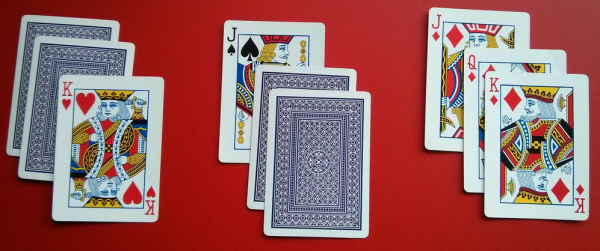# You're reading: Posts By Dave Wilding

### Discovering integer sequences by dealing cardsLet’s play a game:

1. Imagine you have some playing cards. Of course if you actually have some cards you don’t need to imagine!
2. Pick your favourite natural number $n$ and put a deck of $n$ cards in front of you. Then repeat the next step until the deck is empty.
3. Take $2$ cards from the top of the deck and throw them away, or just take $1$ card from the top and throw it away. The choice is yours.

If you pick a small $n$, such as $n=3$, it’s pretty easy to see how this game is going to play out. Choosing to throw away $2$ cards the first time means you’re then forced to throw away $1$ card the next time, but only throwing away $1$ card the first time leaves you with a choice of what to throw away the next time. So for $n=3$ there are exactly $3$ different ways to play the game: throw $2$ then $1$, throw $1$ then $2$, or throw $1$ then $1$ then $1$.

Now, here comes the big question. How does the number of different ways to play this game depend on the size of the starting deck? Or in other words, what integer sequence $a_0$, $a_1$, $a_2$, $a_3$, $a_4$, … do we get if $a_n$ represents the number of different ways to play the game with a deck of $n$ cards? (We already know that $a_3=3$.)